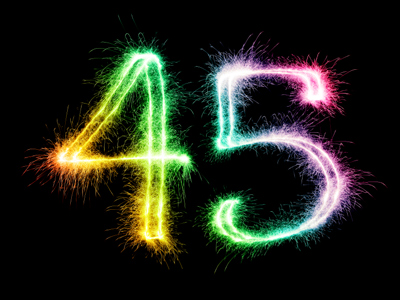If you share 45 between 5, you will get 9.

# Multiplication and Division (Year 3)

Multiplication and division are two key elements of Maths which form an integral part of the subject in KS2. In Year Three children are encouraged to learn their times tables as this will help when multiplying or dividing numbers. Children will be taught other strategies to use as well, for example, simplifying problems to make them easier. They will also be expected to know some of the words associated with multiplication and division such as double or share.

Multiplication and division are easier if you know your times tables. You can practise them by playing our KS2 Times Tables Quizzes. However you still need other strategies to multiply numbers and to divide them. Some problems can be made simpler, for example to multiply 7 by 9 just multiply it by 10 and take 7 away. That makes it much easier to work out.

Take this quiz to find out how good your strategies are when multiplying or dividing numbers.

1.
A tower is 4 bricks tall; how many bricks in a tower 3 times taller?
1
3
12
15
4 x 3 = 12
2.
What number do I get if I share 45 between 5?
4
5
9
10
Share is another way of saying divide
3.
Which number sentence is incorrect?
12 x 4 = 48
4 x 12 = 48
48 ÷ 12 = 4
12 ÷ 4 = 48
12 ÷ 4 = 3 not 48!
4.
How many 6s in 18?
2
3
5
6
18 ÷ 6 = 3
5.
What happens when a number is multiplied by 1?
The number is doubled
The number does not change
The number is halved
The digit moves one place to the right
A number does not change when it is divided by 1 either
6.
What number do you get if you double 18?
36
38
40
42
Remember that double is the same as multiply by 2
7.
30 x 4 = ?
12
60
120
150
If you know 3 x 4 is 12 then just multiply by 10
8.
500 ÷ 10 = ?
5
50
250
5,000
To divide a number by 10 move the decimal point one place to the left
9.
How do we calculate double a number?
Multiply by 10
Divide by 2
Multiply by 2
Divide by 3
Double means the same as x 2
10.
What number do you get if you double 35?
60
70
80
90
35 x 2 = 70